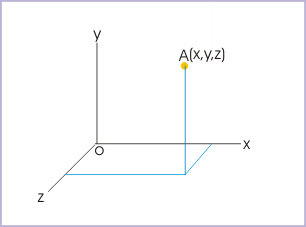# Motion  (Page 2/4)

 Page 2 / 4

The requirement of an observer in both identifying and quantifying motion brings about new dimensions to the understanding of motion. Notably, the motion of a body and its measurement is found to be influenced by the state of motion of the observer itself and hence by the state of motion of the attached frame of reference. As such, a given motion is evaluated differently by different observers (system of references).

Two observers in the same state of motion, such as two persons standing on the platform, perceive the motion of a passing train in exactly same manner. On the other hand, the passenger in a speeding train finds that the other train crossing it on the parallel track in opposite direction has the combined speed of the two trains $\left({v}_{1}+{v}_{1}\right)$ . The observer on the ground, however, find them running at their individual speeds ${v}_{1}$ and ${v}_{2}$ .

From the discussion above, it is clear that motion of an object is an attribute, which can not be stated in absolute term; but it is a kind of attribute that results from the interaction of the motions of the both object and observer (frame of reference).

## Frame of reference and observer

Frame of reference is a mathematical construct to specify position or location of a point object in space. Basically, frame of reference is a coordinate system. There are plenty of coordinate systems in use, but the Cartesian coordinate system, comprising of three mutually perpendicular axes, is most common. A point in three dimensional space is defined by three values of coordinates i.e. x,y and z in the Cartesian system as shown in the figure below. We shall learn about few more useful coordinate systems in next module titled " Coordinate systems in physics ".A point in three dimensional space is defined by three values of coordinates

We need to be specific in our understanding of the role of the observer and its relation with frame of reference. Observation of motion is considered an human endeavor. But motion of an object is described in reference of both human and non-human bodies like clouds, rivers, poles, moon etc. From the point of view of the study of motion, we treat reference bodies capable to make observations, which is essentially a human like function. As such, it is helpful to imagine ourselves attached to the reference system, making observations. It is essentially a notional endeavor to consider that the measurements are what an observer in that frame of reference would make, had the observer with the capability to measure was actually present there.

Earth is our natural abode and we identify all non-moving ground observers equivalent and at rest with the earth. For other moving systems, we need to specify position and determine motion by virtually (in imagination) transposing ourselves to the frame of reference we are considering.

Take the case of observations about the motion of an aircraft made by two observers one at a ground and another attached to the cloud moving at certain speed. For the observer on the ground, the aircraft is moving at a speed of ,say, 1000 km/hr.

pls explain what is dimension of 1in length and -1 in time ,what's is there difference between them
what are scalars
show that 1w= 10^7ergs^-1
what's lamin's theorems and it's mathematics representative
if the wavelength is double,what is the frequency of the wave
What are the system of units
A stone propelled from a catapult with a speed of 50ms-1 attains a height of 100m. Calculate the time of flight, calculate the angle of projection, calculate the range attained
58asagravitasnal firce
Amar
water boil at 100 and why
what is upper limit of speed
what temperature is 0 k
Riya
0k is the lower limit of the themordynamic scale which is equalt to -273 In celcius scale
Mustapha
How MKS system is the subset of SI system?
which colour has the shortest wavelength in the white light spectrum
if x=a-b, a=5.8cm b=3.22 cm find percentage error in x
x=5.8-3.22 x=2.58
what is the definition of resolution of forcesBy OpenStaxBy OpenStaxBy OpenStaxBy Stephen VoronBy Madison ChristianBy Robert MurphyBy Mariah HauptmanBy Brooke DelaneyBy JavaChamp TeamBy Rachel Carlisle